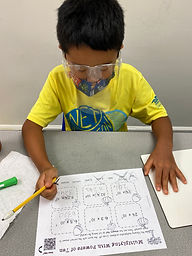Ms. Kylie

Target 1​

Lesson Type:

Continuation

Number Operation

:

Computation

Express powers of ten, using exponents.

1:

Understand that when multiplying a whole number by a power of ten, the product will have an increased number of zeros, that has a direct relationship to the exponent.

2:

Understand that multiplying and dividing by a power of ten relates to the placement of a decimal point in a number.

6th

Vocabulary:

Power of 10, Exponent, Place Value

Activities:

• Students learned when multiplying decimals by powers of 10 the number is increasing by moving the decimal point over to the right by the amount of zeros.
• Students practiced multiplying decimals by a power of 10Home Exploration

Guiding Questions:Absent Students:

Target 2

:

1:

Define translational symmetry.

2:

Understand a translation as a movement (shift or slide) of a figure in a specified direction through a specified distance without any rotation or reflection.

6th

Vocabulary:

Translational symmetry, Coordinate plane

Activities:

• Students learned translational symmetry is moving a 2D shape in a specific direction for a specific distance.
• Students graphed a 2D shape on a coordinate plane and practiced translating the shape by the given distance from a specific point.Home Exploration

Guiding Questions:Target 3

:

Vocabulary:

Activities:Home Exploration